Variational EM inference of Stochastic Block Models indexed by block number from a partially observed network.

estimateMissSBM(
vBlocks,
sampling,
covariates = list(),
control = list()
)

## Arguments

adjacencyMatrix The N x N adjacency matrix of the network data. If adjacencyMatrix is symmetric, we assume an undirected network with no loop; otherwise the network is assumed to be directed. The vector of number of blocks considered in the collection. The model used to described the process that originates the missing data: MAR designs ("dyad", "node","covar-dyad","covar-node","snowball") and MNAR designs ("double-standard", "block-dyad", "block-node" , "degree") are available. See details. An optional list with M entries (the M covariates). If the covariates are node-centered, each entry of covariates must be a size-N vector; if the covariates are dyad-centered, each entry of covariates must be N x N matrix. a list of parameters controlling advanced features. See details.

## Value

Returns an R6 object with class missSBM_collection.

## Details

Internal functions use future_lapply, so set your plan to 'multisession' or 'multicore' to use several cores/workers. The list of parameters control tunes more advanced features, such as the initialization, how covariates are handled in the model, and the variational EM algorithm:

• "useCov": logical. If covariates is not null, should they be used for the for the SBM inference (or just for the sampling)? Default is TRUE.

• "clusterInit": Initial method for clustering: either a character ("spectral") or a list with length(vBlocks) vectors, each with size ncol(adjacencyMatrix), providing a user-defined clustering. Default is "spectral".

• "similarity": An R x R -> R function to compute similarities between node covariates. Default is missSBM:::l1_similarity, that is, -abs(x-y). Only relevant when the covariates are node-centered (i.e. covariates is a list of size-N vectors).

• "threshold": V-EM algorithm stops stop when an optimization step changes the objective function or the parameters by less than threshold. Default is 1e-2.

• "maxIter": V-EM algorithm stops when the number of iteration exceeds maxIter. Default is 50.

• "fixPointIter": number of fix-point iterations in the V-E step. Default is 3.

• "exploration": character indicating the kind of exploration used among "forward", "backward", "both" or "none". Default is "both".

• "iterates": integer for the number of iterations during exploration. Only relevant when exploration is different from "none". Default is 1.

• "trace": logical for verbosity. Default is TRUE.

The different sampling designs are split into two families in which we find dyad-centered and node-centered samplings. See doi: 10.1080/01621459.2018.1562934 for a complete description.

• Missing at Random (MAR)

• "node": parameter = p = Prob(Node i is observed)

• "covar-dyad": parameter = beta in R^M, such that Prob(Dyad (i,j) is observed) = logistic(parameter' covarArray (i,j, .))

• "covar-node": parameter = nu in R^M such that Prob(Node i is observed) = logistic(parameter' covarMatrix (i,)

• "snowball": parameter = number of waves with Prob(Node i is observed in the 1st wave)

• Missing Not At Random (MNAR)

• "double-standard": parameter = (p0,p1) with p0 = Prob(Dyad (i,j) is observed | the dyad is equal to 0), p1 = Prob(Dyad (i,j) is observed | the dyad is equal to 1)

• "block-node": parameter = c(p(1),...,p(Q)) and p(q) = Prob(Node i is observed | node i is in cluster q)

• "block-dyad": parameter = c(p(1,1),...,p(Q,Q)) and p(q,l) = Prob(Edge (i,j) is observed | node i is in cluster q and node j is in cluster l)

• "degree": parameter = c(a,b) and logit(a+b*degree(i)) = Prob(Node i is observed | Degree(i))

observeNetwork, missSBM_collection and missSBM_fit.

## Examples

## SBM parameters
N <- 100 # number of nodes
Q <- 3   # number of clusters
pi <- rep(1,Q)/Q     # block proportion
theta <- list(mean = diag(.45,Q) + .05 ) # connectivity matrix

## Sampling parameters
samplingParameters <- .75 # the sampling rate
sampling  <- "dyad"      # the sampling design

## generate a undirected binary SBM with no covariate
sbm <- sbm::sampleSimpleSBM(N, pi, theta)

## Uncomment to set parallel computing with future
## future::plan("multicore", workers = 2)

## Sample some dyads data + Infer SBM with missing data
collection <-
observeNetwork(sbm$networkData, sampling, samplingParameters) %>% estimateMissSBM(vBlocks = 1:5, sampling = sampling) #> #> #> Adjusting Variational EM for Stochastic Block Model #> #> Imputation assumes a 'dyad' network-sampling process #> #> Initialization of 5 model(s). #> Performing VEM inference #> Model with 5 blocks. Model with 1 blocks. Model with 4 blocks. Model with 3 blocks. Model with 2 blocks. #> Looking for better solutions #> Pass 1 Going forward ++++ Pass 1 Going backward ++++ plot(collection, "monitoring")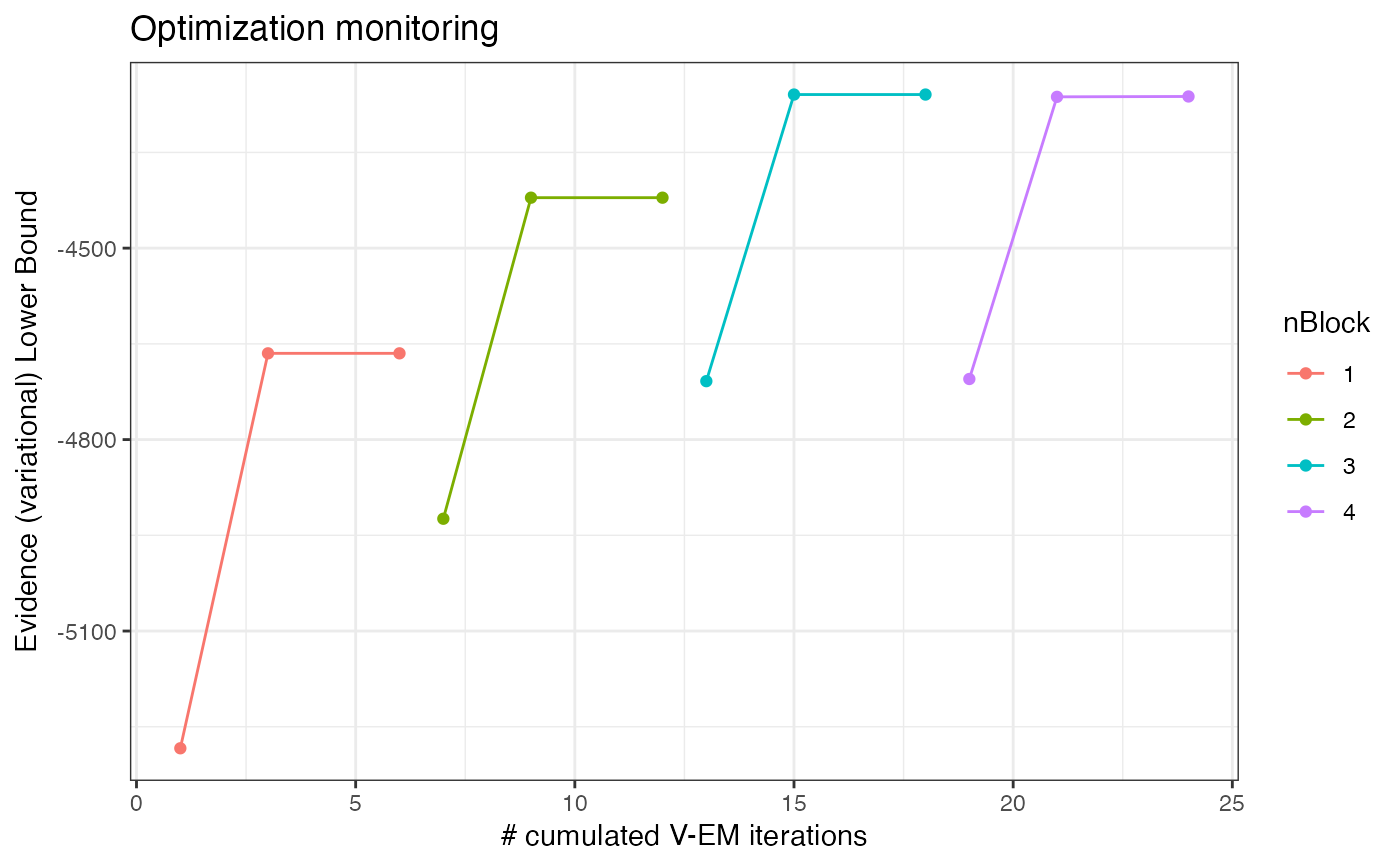plot(collection, "icl")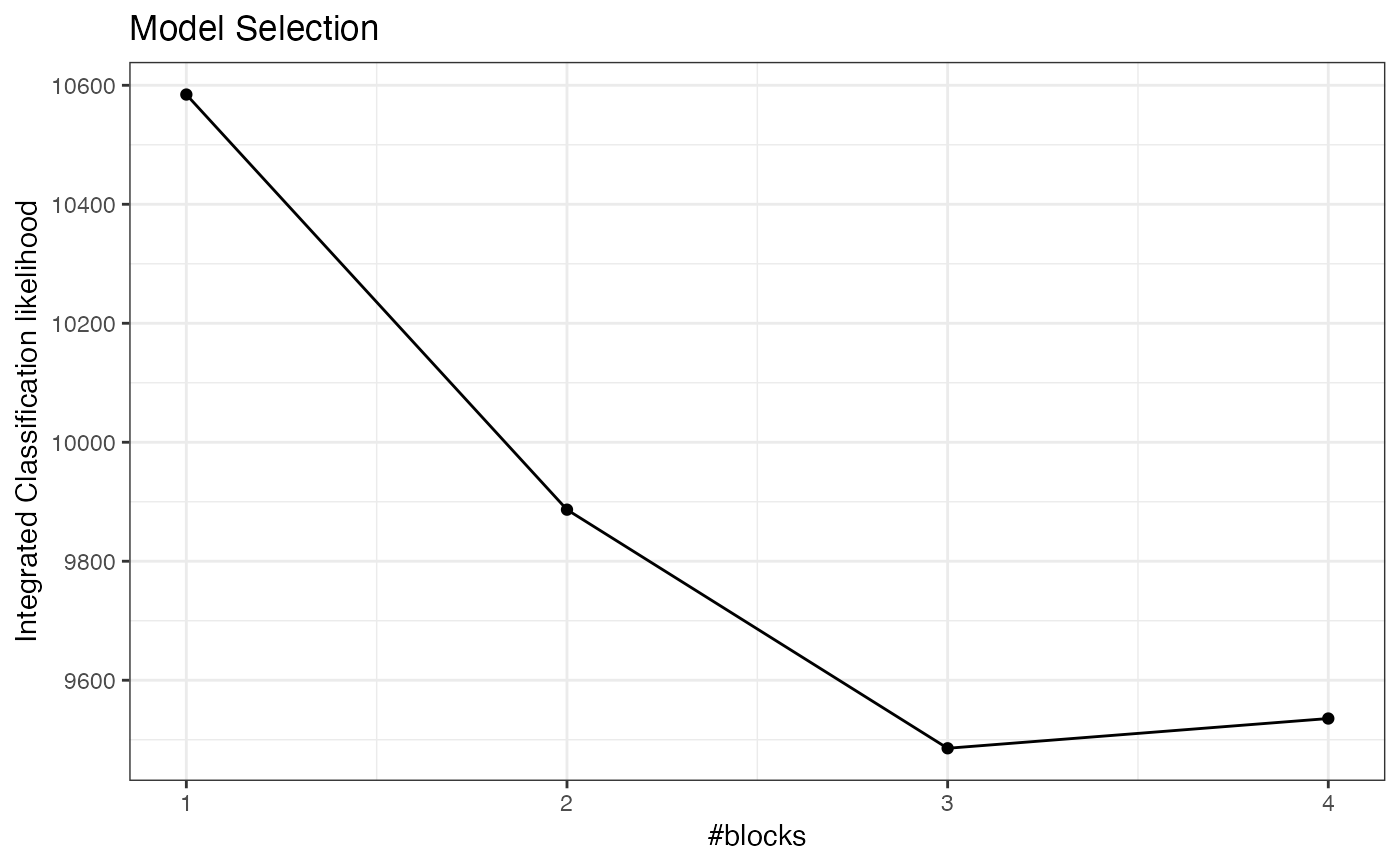collection$ICL
#>  10584.442  9886.688  9485.626  9534.831  9587.181coef(collection$bestModel$fittedSBM, "connectivity")
#> $mean #> [,1] [,2] [,3] #> [1,] 0.50247919 0.05509355 0.05696972 #> [2,] 0.05509355 0.50953677 0.05164995 #> [3,] 0.05696972 0.05164995 0.53003541 #> myModel <- collection$bestModel
plot(myModel, "expected")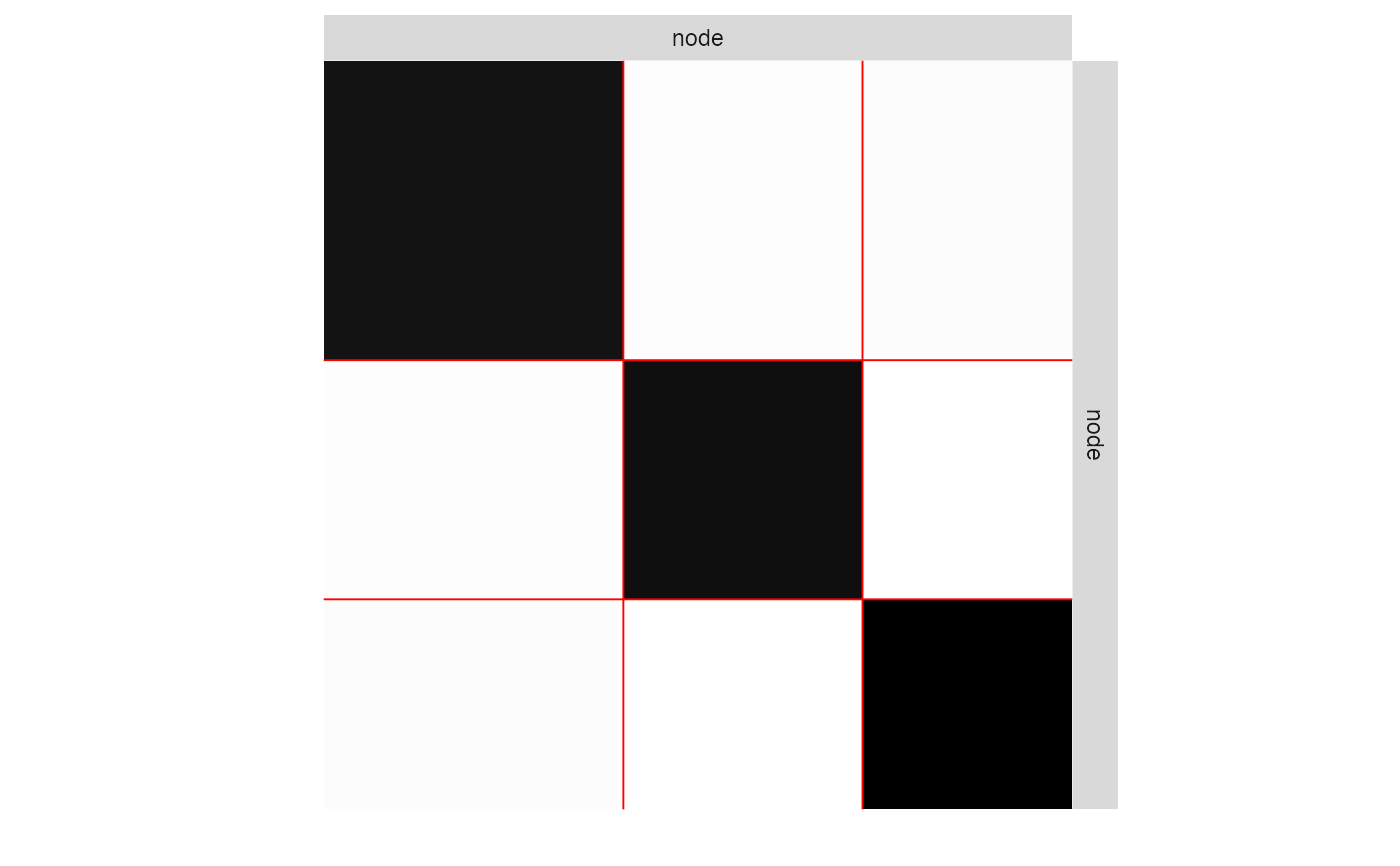plot(myModel, "imputed")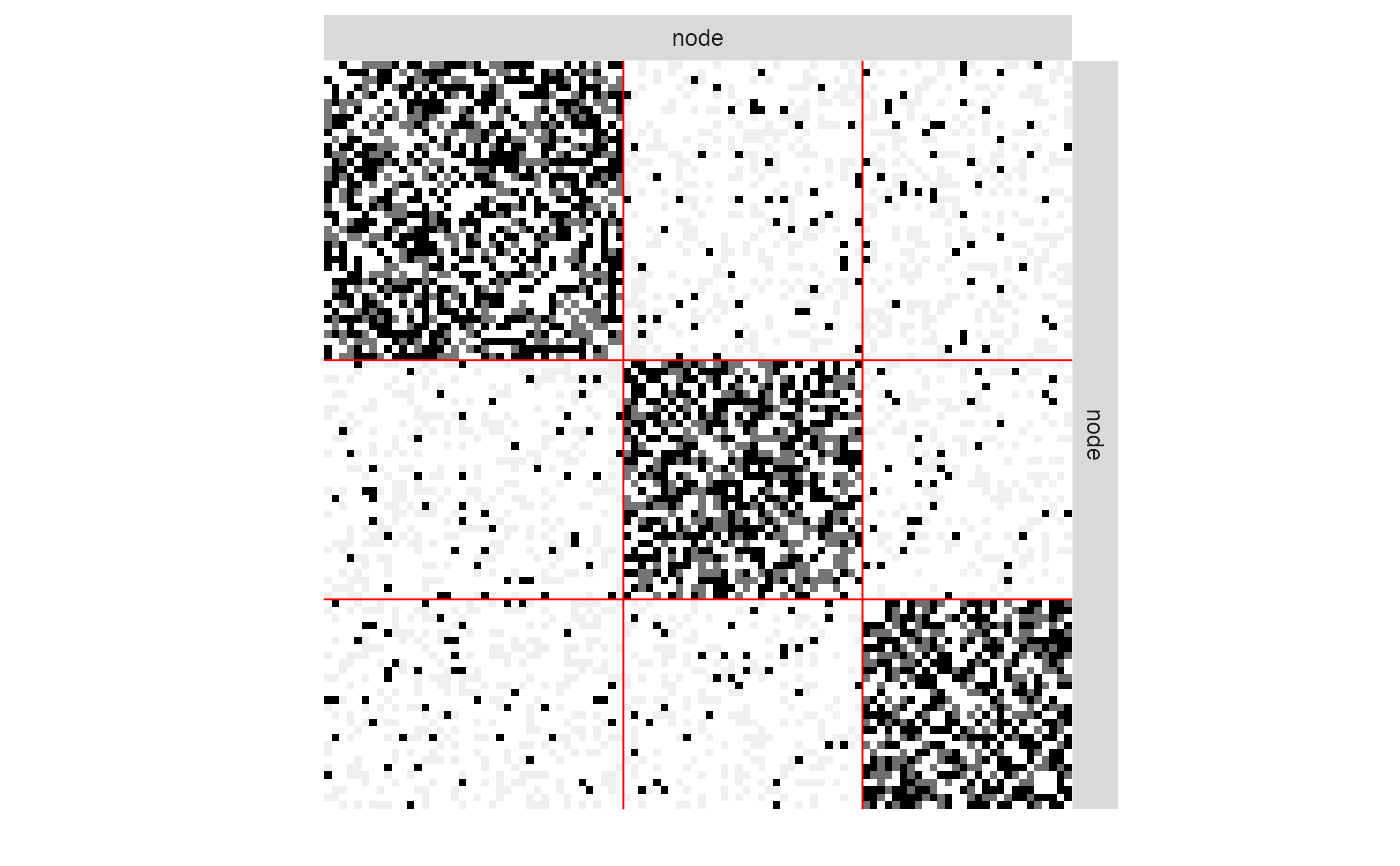plot(myModel, "meso")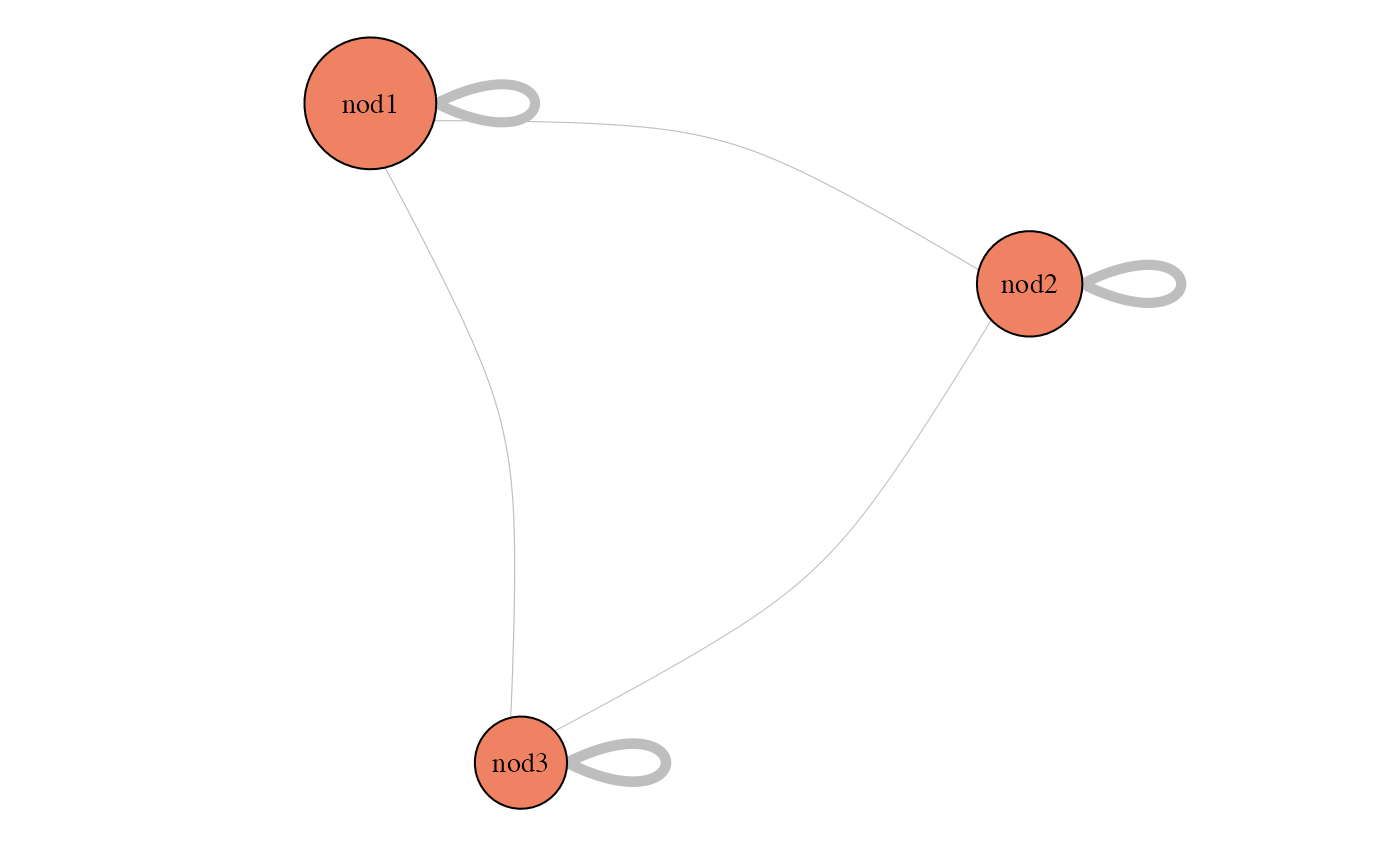coef(myModel, "sampling")
#>  0.7553535coef(myModel, "connectivity")
#> \$mean
#>            [,1]       [,2]       [,3]
#> [1,] 0.50247919 0.05509355 0.05696972
#> [2,] 0.05509355 0.50953677 0.05164995
#> [3,] 0.05696972 0.05164995 0.53003541
#> predict(myModel)[1:5, 1:5]
#> 5 x 5 sparse Matrix of class "dgCMatrix"
#>
#> [1,] .          . 0.05164995 .          .
#> [2,] .          . 1.00000000 1.00000000 .
#> [3,] 0.05164995 1 .          1.00000000 .
#> [4,] .          1 1.00000000 .          0.05509355
#> [5,] .          . .          0.05509355 .